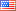# Revision history [back]

I have solved the program with the following modification. But comparatively this takes a lot of time for larger $n$ as the same loop runs twice. Can we do it by running the loop only once?

def xyz(n): m=max([a.det() for a in myfunction(n)]) for A in myfunction(n): if A.det()==m: print A

I have solved the program problem with the following modification. But comparatively this takes a lot of time for larger $n$ as the same loop runs twice. Can we do it by running the loop only once?

def xyz(n): m=max([a.det() for a in myfunction(n)]) for A in myfunction(n): if A.det()==m: print A3 No.3 RevisionJohn Palmieri7648 ●18 ●66 ●166 http://www.math.washin...

I have solved the problem with the following modification. But comparatively this takes a lot of time for larger $n$ as the same loop runs twice. Can we do it by running the loop only once?

def xyz(n):
m=max([a.det() for a in myfunction(n)])
for A in myfunction(n):
if A.det()==m:
print AA